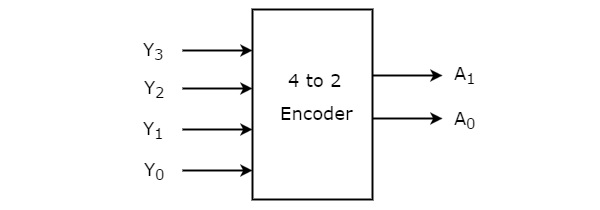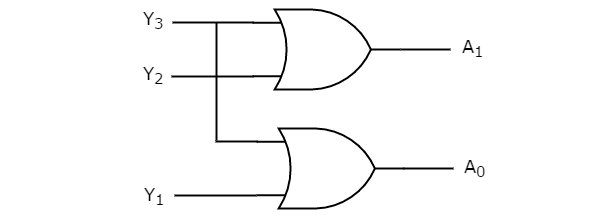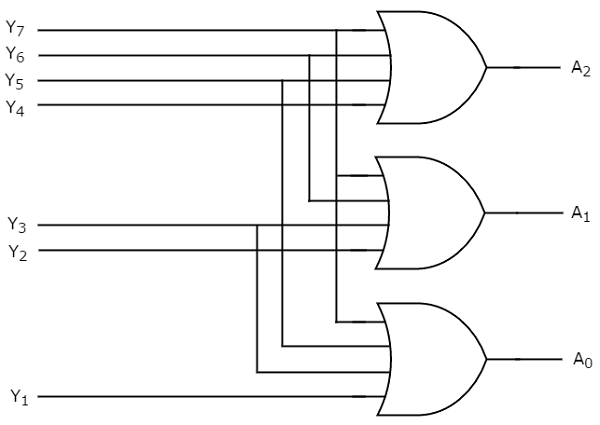Goseeko blog# What is Encoder?￼

The reverse operation of the Decoder is performed by an encoder, which is a combinational circuit. There are a total of 2n input lines and ‘n’ output lines. It will generate a binary code that is equivalent to the active High input. As a result, the encoder uses ‘n’ bits to encode 2n input lines. In encoders, representing the enable signal is optional.

## 4 to 2 line Encoder:

There are four inputs (Y0, Y1, Y2, and Y3) and two outputs (A0 and A1) in the 4 to 2 line encoder. In addition, To get the respective binary code on the output side, one input line at a time is set to true in a 4-input line. The 4 to 2 line encoder’s block diagram and truth table are shown below.Truth table

The terms A0 and A1 are logically expressed as follows:

A1=Y3+Y2

A0=Y3+Y1

Circuit Diagram

Two input OR gates can be used to implement the aforementioned two Boolean functions. Further, The 4 to 2 encoder circuit diagram is given in the graphic below.## Octal to Binary Encoder

The octal to binary encoder has eight inputs, ranging from Y7 to Y0, and three outputs, A2, A1, and A0. The 8 to 3 encoder is the same as the octal to binary encoder. The following graphic depicts the block diagram of an octal to binary encoder.

Only one of these eight inputs can be ‘1’ at any given time in order to obtain the binary code. The octal to binary encoder’s truth table is presented below.

Truth Table

The following is the logical expression of the terms A0, A1, and A2:

A2=Y4+Y5+Y6+Y7

A1=Y2+Y3+Y6+Y7

A0=Y7+Y5+Y3+Y1

Circuit Diagram

The following is the logical circuit for the above expressions:## Uses of Encoder

In all digital systems, these systems are relatively simple to operate.

To convert a decimal number to a binary number, encoders are employed. The goal is to complete a binary operation like addition, subtraction, multiplication, and so on.